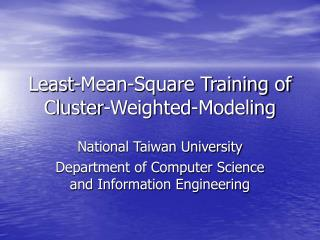Download PresentationLeast-Mean-Square Training of Cluster-Weighted-ModelingLeast-Mean-Square Training of Cluster-Weighted-Modeling - PowerPoint PPT Presentation

Download PresentationLeast-Mean-Square Training of Cluster-Weighted-Modeling
An Image/Link below is provided (as is) to download presentation

Download Policy: Content on the Website is provided to you AS IS for your information and personal use and may not be sold / licensed / shared on other websites without getting consent from its author. While downloading, if for some reason you are not able to download a presentation, the publisher may have deleted the file from their server.

- - - - - - - - - - - - - - - - - - - - - - - - - - - E N D - - - - - - - - - - - - - - - - - - - - - - - - - - -
Presentation Transcript

1. Least-Mean-Square Training of Cluster-Weighted-Modeling National Taiwan University Department of Computer Science and Information Engineering

2. Outline • Introduction of CWM • Least-Mean-Square Training of CWM • Experiments • Summary • Future work • Q&A

3. Cluster-Weighted Modeling (CWM) • CWM is a supervised learning model which are based on the joint probability density estimation of a set of input and output (target) data. • The joint probability is expended into clusters which describe local subspaces well. Each local Gaussian expert can have its own local function (constant, linear or quadratic function). • The global (nonlinear) model can be constructed by combining all the local models. • The resulting model has transparent local structures and meaningful parameters.

4. Architecture • sdff

5. Prediction calculation • Conditional forecast: The expected output given the input. • Conditional error (output uncertainty): The expected output covariance given the input

6. Training (EM Algorithm) • Objective function: Log-likelihood function • Initialize cluster means (k-means), variances (maximal range for each dimension). Initialize =1/M. M: Predetermined number of clusters. • E-step: Evaluate the posterior probability • M-step: Update clusters means Update prior probability

7. M-step ( Cont.) • Define cluster-weighted expectation • Update cluster-weighted covariance matrices • Update cluster parameters which maximizes the data likelihood where • Update output covariance matrices

8. Least-Mean-Square Training of CWM • To train CWM’s model parameters from a least-squared perspective. • Minimizing squared error function of CWM’s training result to find another solution which can have a better accuracy. • To find another solution when CWM is trapped in local minima. • Applying supervised selection of cluster centers instead of unsupervised method.

9. LMS Learning Algorithm The instantaneous error produced by sample n is The prediction formula is Using softmax function to constrain prior probability to have value between 0 and 1 and their summation equal to 1.

10. LMS Learning Algorithm (cont.) • The derivation of gradients:

11. LMS CWM Learning Algorithm • Initialization: Initialize Using CWM’s training result. Initialize Iterate until convergence: For n=1:N Estimate error Estimate gradients Update End E-step: M-step:

12. Simple Demo • cwm1d • cwmprdemo • cwm2d • lms1d

13. Experiments • A simple Sin function. • LMS-CWM has a better interpolation result.

14. Mackey-Glass Chaotic Time Series Prediction • 1000 data points. We take the first 500 points as training set, the last 500 points are chosen as test set. • Single-step prediction • Input: [s(t),s(t-6),s(t-12),s(t-18)] • Output: s(t+85) • Local linear model • Number of clusters: 30

15. Results (1) CWM LMS-CWM

16. Results (2) • Learning curve CWM LMS CWM

17. Local Minima • The initial locations of four clusters. The initial locations of four clusters The resulting centers’ locations after each training session of CWM and LMS-CWM.

18. Summary • A LMS learning method for CWM is presented. • May lose the benefits of data density estimation and characterizing data. • Provides an alternative training option. • Parameters can be trained by EM and LMS alternatively. • Combine both advantages of EM and LMS learning. • LMS-CWM learning can be viewed as a refinement to CWM if only prediction accuracy is our main concern.

19. Future work • Regularization. • Comparison between different models (from theoretical, performance point of views)

20. Q&A Thank You!